## Classification

#### Classification

Direction: In each problem, out of the five figures marked (a), (b), (c), (d) and (e), four are similar in a certain manner. However one figure is not like the other four. Choose the figure which is different from the rest.

1. Choose the figure which is different from the rest.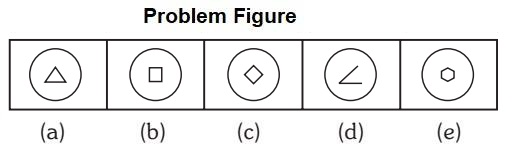1. All inner figures are enclosed one except (d)

##### Correct Option: D

All inner figures are enclosed one except (d)

1. Choose the figure which is different from the rest.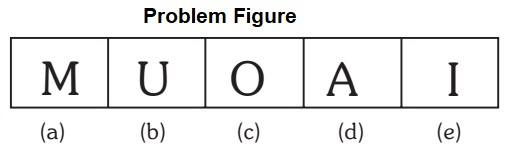1. One letter is only consonant.

##### Correct Option: A

M is only consonant, rest are vowel.

1. Choose the figure which is different from the rest.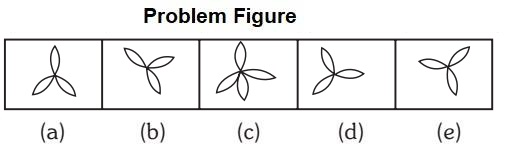1. All has three petals except one.

##### Correct Option: C

Rest all has three petals except (c) which has four petals.

1. Choose the figure which is different from the rest.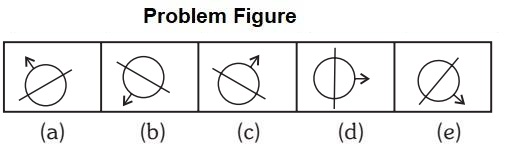1. Arrows are moving anti clock wise direction.

##### Correct Option: B

Arrows are moving anti clock wise direction by 45 degree.

1. Choose the figure which is different from the rest.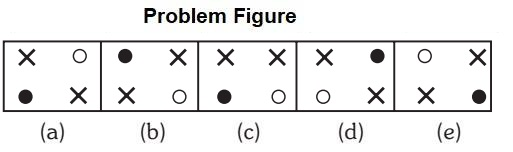1. All figures are diagonally opposite direction except one.

##### Correct Option: C

Except (c) rest elements of figures are diagonally opposite direction.